Next: Orbifold Compactifications Up: SUPERSTRING PHENOMENOLOGY BEFORE 1995 Previous: String Model Building

## Toroidal Compactifications

In order to construct string models in less than 10D as well as to understand the heterotic string construction, we need to consider the simplest compactifications which correspond to the extra dimensions being circles and their higher dimensional generalization.

Let us first see the case of a circle. This means that the 10D space is represented by flat 9D spacetime times a circle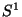. We know that a circle is just the real line identifying all the numbers differing by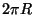, where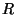is the radius of the circle. So the only difference with the flat space discussed above are the boundary conditions. The solution of the wave equations are now as in (4). But now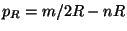and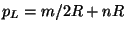,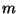is an integer reflecting the fact that the momentum in the compact direction has to be quantized in order to get single-valued wave function. The integer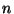however refers to the fact that the string can wind around several times in the compact dimension and is thus named the winding number'. The mass formula is then: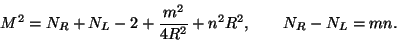(6)

This shows several interesting facts. First, for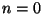and varying, we obtain an infinite tower of massive states with masses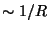; these are the standard momentum states' of Kaluza-Klein compactifications in field theory. In particular the massless states with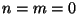and one oscillator in the compact direction are vector fields in the extra dimensions giving rise to a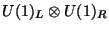Kaluza-Klein gauge symmetry. The states with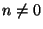are the winding states and are purely stringy; they represent string states winding around the circle, they have mass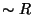. Second, there are special values ofandwhich can give rise to extra massless states. In particular for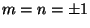we can see that at the special radius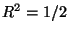in units of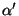, there are massless states with a single oscillator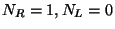corresponding to massless vectors which in this case generate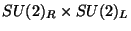. This means that the special point in the moduli space' of the circleis a point of enhanced symmetry. The original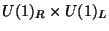Kaluza-Klein symmetry of compactification on a circle gets enhanced to. This is a very stringy effect because it depends crucially on the existence of winding modes (). The third interesting fact about this compactification is that the spectrum is invariant under the following duality' transformations :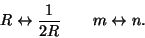(7)

This is also a stringy property. It exchanges small with large distances but at the same time it exchanges momentum (Kaluza-Klein) states with winding states. This symmetry can be shown to hold not only for the spectrum but also for the interactions and therefore it is an exact symmetry of string perturbation theory.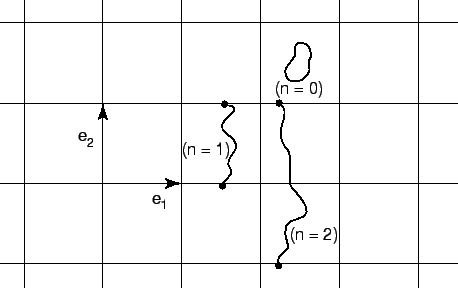Let us now extend the compactification to two dimensions, i.e., the 26D spacetime is the product of flat 24D spacetime and a 2D generalization of a circle, the torus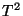. Again the only difference with flat space is the boundary conditions. The two compact dimensions are identified by vectors of a 2D lattice, defining the torus. Out of the three independent components of the compactified metric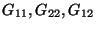and the single component of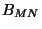namely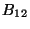we can build two complex moduli' fields: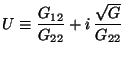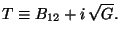(8)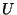is the standard modular parameter of any geometrical 2D torus and it is usually identified as the complex structure' modulus.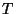is the Kähler structure' modulus (sinceis a complex Kähler space) and its imaginary part measures the overall size of the torus, since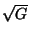is the determinant of the 2D metric. It plays the same role asdid for the 1D circle. In terms ofandwe can write the left- and right-moving momenta as: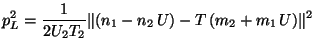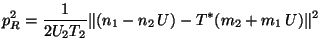(9)

The mass forula, depending on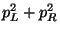, again shows that there are enhanced symmetry points for special values ofand. It also shows the following symmetries: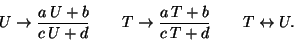(10)

Where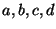are integers satisfying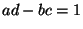. The first transformation is the standard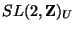modular' symmetry of 2D tori and is independent of string theory; it is purely geometric. The second transformation is a stringy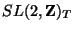named-duality and it is a generalization of (7) for the 2D case. Again this is a symmetry as long as we also transform momenta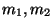with winding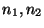. The third symmetry exchanges the complex structurewith the Kähler structureand it is called `mirror symmetry'. Ifandeach parametrize a complex plane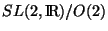, the duality symmetry implies that they can only live in the fundamental domain defined by all the points of the product of complex spaces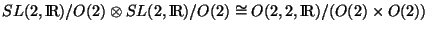identified under the duality group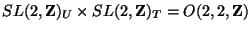.

This is the situation that gets generalized to higher dimensions. In general, compactification on a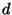-dimensional torus has the moduli space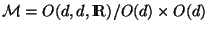with points identified under the duality group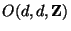. For the heterotic string with 16 extra left moving coordinates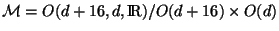with a similar modification to the duality group. The left- and right- moving momenta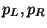live on an even, selfdual lattice of signature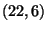, which is usually called the Narain lattice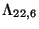. This generalizes the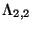lattice defined by the integers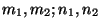of eq. (9).

We can easily verify in this case that the dimension of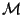is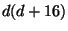corresponding to the number of independent components of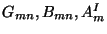with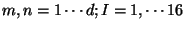. For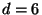we have a 4D string model with a moduli space of dimension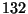. To this we have to add the dilaton field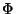which, together with the spacetime components of the antisymmetric tensor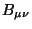, can be combined into a new modular parameter: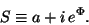(11)

Here the axion field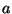is defined as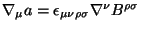.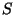parametrizes again a coset. It is then natural to believe there is also a duality symmetry for the fieldof the type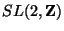; by analogy with the situation forand. Such a symmetry was proposed in ref. and it has received a lot of attention recently. If true it may have far reaching consequences since (similar to equation (7)) it relates strong to weak string coupling.Next: Orbifold Compactifications Up: SUPERSTRING PHENOMENOLOGY BEFORE 1995 Previous: String Model Building
root 2001-01-22